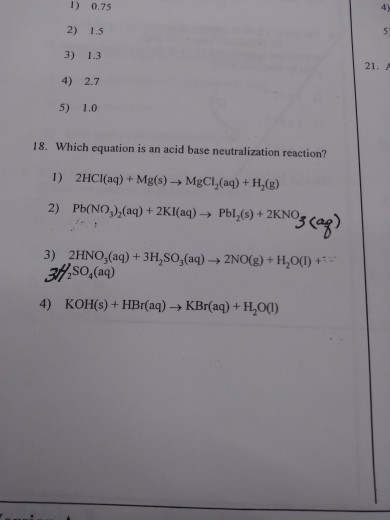# Question & Answer: Which equation is an acid base neutralization reaction? 1) 2HCl(aq) + Mg(s) rightarrow…..Which equation is an acid base neutralization reaction? 1) 2HCl(aq) + Mg(s) rightarrow MgCl_2 (aq) + H_2 (g) 2) Pb(NO_3)_2 (aq) + 2Kl(aq) rightarrow Pbl_2 (s) + 2KNO 3) 2HNO_3 (aq) + 3H_2SO_3 (aq) rightarrow 2NO(g) + H_2O(l) + SO_4 (aq) 4) KOH(s) + HBr(aq) rightarrow KBr(aq) + H_2O(l)